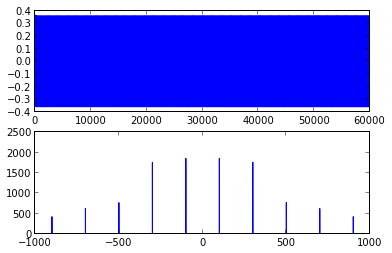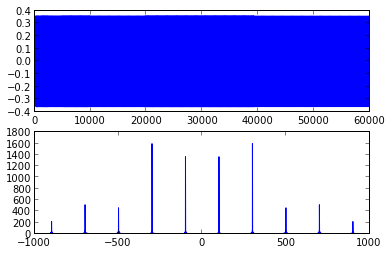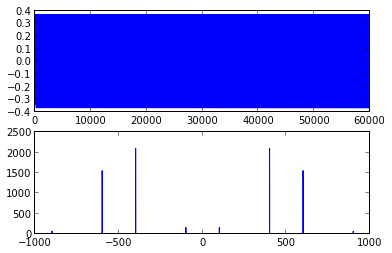In :
import scipy.signal
import math

def plotspec(x, Ts):
fig = figure()
ax1.plot(x)

q = fft.fft(x)
ax2.plot(fft.fftfreq(len(x), Ts), abs
(q))

def plotfilter(b, Ts):
figure()
time = 3.0
x = random.uniform(-1.0,1.0, time/Ts)
y = scipy.signal.lfilter(b,1,x)
plotspec(y, Ts)

def genlpf(ntaps, topf, Ts):
q = topf/(1/Ts)
b = scipy.signal.remez(ntaps, [0, q, 1.08*q, 0.5], [1,0])
return b

def genhpf(ntaps, bottomf, Ts):
q = bottomf/(1/Ts)
b = scipy.signal.remez(ntaps, [0, q, 1.08*q, 0.5], [0,1])
return b

def genbandpassf(ntaps, bottomf, topf, Ts):
q = bottomf/(1/Ts)
r = topf/(1/Ts)

b = scipy.signal.remez(ntaps, [0,q*0.98,q,r,r*1.02,0.5], [0,1,0])
return b


6.3. Create a simulation of a sampling based modulator that takes a signal with abndwidth 100Hz and transforms it into the same signal centered at 5kHz.

In :
# Create a signal with BW=100Hz:

Ts = 1.0/20000.0
time = 3.0

b = genlpf(100, 100, Ts)
n = 0.25*random.normal(0,1,time/Ts)
plotspec(n, Ts)

nf = scipy.signal.lfilter(b,1, n)
plotspec(nf, Ts)

def resample(w, fc, Ts):
Tc = 1.0/fc

# Need to find the "nearest" sample points at which to downsample
lw = len(w)
stepsize = Tc/Ts

# Build a pulse train at these points
pulse_train = linspace(0,0,lw)

sampleno = 0.0

while(sampleno < lw):
sampleno += stepsize
if (round(sampleno) < lw):
pulse_train[round(sampleno)] = 1.0

sp = w*pulse_train

return sp

def sample_modulate(w, fc, Ts):
sp = resample(w, fc, Ts)

b2 = genbandpassf(100, fc/2, 3.0*fc/2.0, Ts)
return scipy.signal.lfilter(b2, 1, sp)

w = sin(linspace(0, time, time/Ts)*2*pi*100)
y = sample_modulate(w, 5000.0, Ts)
plotspec(y, Ts)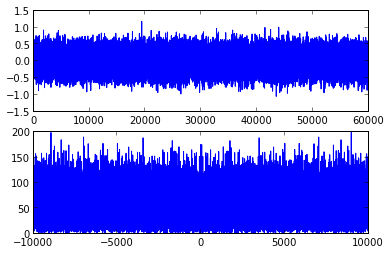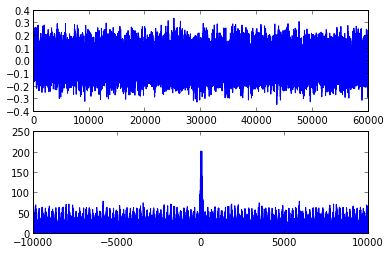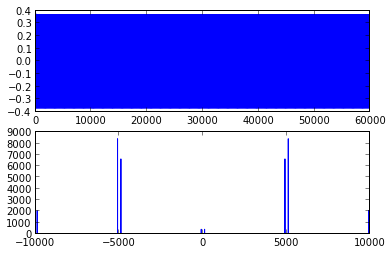6.4. Implement the procedure diagrammed in Figure 6.5. Comment on the choice of sampling rates. how have you specified the LPF?

In :
def sample_demodulate(s, fc, fcutoff, Ts):
b = genlpf(100, fcutoff, Ts)
w = resample(s, fc, Ts)
m = scipy.signal.lfilter(w, 1, b)
return w

w = sin(linspace(0, time, time/Ts)*2*pi*100)
plotspec(w, Ts)
xlim((-200, 200))

y = sample_modulate(w, 5000.0, Ts)
plotspec(y, Ts)

s = sample_demodulate(y, 5000.0, 1.0, Ts)
plotspec(s, Ts)
xlim((-200,200))

Out:
(-200, 200)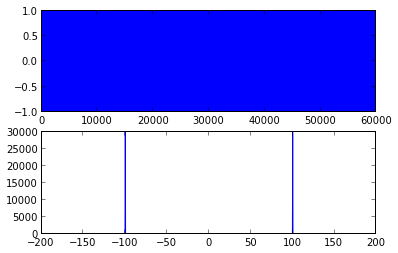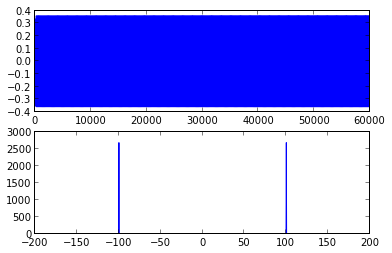6.5. Using your code from Problem 6.4, examine the effect of"incorrect" sampling rates by demodulating with fs+gamma instead of fs. This is analogous to the problem that occurs in cosine mixing demodulation where the frequenecy is not accurate. Is there an analogy to the phase problem that occurs, wfor instance, with nonzero phi in (5.4)?

In :
def sample_demodulate(s, fc, fcutoff, Ts):
b = genlpf(100, fcutoff, Ts)
w = resample(s, fc, Ts)
m = scipy.signal.lfilter(w, 1, b)
return w

w = sin(linspace(0, time, time/Ts)*2*pi*100)
plotspec(w, Ts)
xlim((-200, 200))

y = sample_modulate(w, 5000.0, Ts)
plotspec(y, Ts)

for gamma in [200, -200, 500]:
s = sample_demodulate(y, 5000.0+gamma, 1.0, Ts)
plotspec(s, Ts)
xlim((-1000,1000))